Hu, K., Wang, B., Shen, Y., Guan, J., and Cai, Y. (2020). "Defect identification method for poplar veneer based on progressive growing generated adversarial network and MASK R-CNN model," BioRes. 15(2), 3041-3052.

#### Abstract

As the main production unit of plywood, the surface defects of veneer seriously affect the quality and grade of plywood. Therefore, a new method for identifying wood defects based on progressive growing generative adversarial network (PGGAN) and the MASK R-CNN model is presented. Poplar veneer was mainly studied in this paper, and its dead knots, live knots, and insect holes were identified and classified. The PGGAN model was used to expand the dataset of wood defect images. A key ideal employed the transfer learning in the base of MASK R-CNN with a classifier layer. Lastly, the trained model was used to identify and classify the veneer defects compared with the back- propagation (BP) neural network, self-organizing map (SOM) neural network, and convolutional neural network (CNN). Experimental results showed that under the same conditions, the algorithm proposed in this paper based on PGGAN and MASK R-CNN and the model obtained through the transfer learning strategy accurately identified the defects of live knots, dead knots, and insect holes. The accuracy of identification was 99.05%, 97.05%, and 99.10%, respectively.

Defect Identification Method for Poplar Veneer Based on Progressive Growing Generated Adversarial Network and MASK R-CNN Model

Kai Hu,a Baojin Wang,a,* Yi Shen,b Jieru Guan,a and Yi Cai a

As the main production unit of plywood, the surface defects of veneer seriously affect the quality and grade of plywood. Therefore, a new method for identifying wood defects based on progressive growing generative adversarial network (PGGAN) and the MASK R-CNN model is presented. Poplar veneer was mainly studied in this paper, and its dead knots, live knots, and insect holes were identified and classified. The PGGAN model was used to expand the dataset of wood defect images. A key ideal employed the transfer learning in the base of MASK R-CNN with a classifier layer. Lastly, the trained model was used to identify and classify the veneer defects compared with the back- propagation (BP) neural network, self-organizing map (SOM) neural network, and convolutional neural network (CNN). Experimental results showed that under the same conditions, the algorithm proposed in this paper based on PGGAN and MASK R-CNN and the model obtained through the transfer learning strategy accurately identified the defects of live knots, dead knots, and insect holes. The accuracy of identification was 99.05%, 97.05%, and 99.10%, respectively.

Keywords: Veneer defects; PGGAN; MASK R-CNN; Identification; Transfer learning

Contact information: a: Faculty of Material Science and Engineering, Nanjing Forestry University, Nanjing 210037, China; b: Zhenjiang Zhongfuma Machinery Co., Ltd., Zhenjiang 212127, China;

* Corresponding author: wangbaojincn@139.com.cn

INTRODUCTION

Veneer defect detection and identification plays an important role in the production process of plywood. The traditional detection method of spot defects on the surface of veneer is manual detection, which has high production cost and low efficiency. The demand of automation production is increasingly urgent (Yang et al. 2006). With the development of artificial intelligence technology, deep learning has achieved positive results in image-based classification and target recognition tasks in recent years (An et al. 2017). However, deep neural networks require large amounts of data; the cost of collecting wood images through machines is high (Viguier et al. 2017). Therefore, the dataset of the classification machine is usually too small to train a deep network. In addition, a lot of manual work is required to mark all the collected images, so deep learning is rarely used in the wood industry (Chang et al. 2018). For the detection and classification of wood defects, scholars put forward a variety of methods. Gu et al. (2009) proposed a tree support vector machine (SVM) to classify four types of wood defects using board images. First, the knot image is divided into three different regions, and then the average pseudo-color feature of each region is obtained by applying ordered statistical filtering. Support vector machine classifier trained with 800 wood knot images has achieved good classification results. The performance evaluation showed that the average classification rate of over 400 sub images is 96.5% and the error frequency is 2.25% (Gu et al. 2009). In 2012, Amir proposed three methods combined with the gray co-occurrence matrix method, local binary mode, and statistical moment when extracting the features of defects, and used principal component analysis (PCA) and linear discriminant analysis (LDA) to reduce the dimension of vectors. Subsequently, Support Vector Machine (SVM) and K-Nearest Neighbor (KNN) were used for classification, and satisfactory results were achieved in the classification of wood defects (Mahram et al. 2012). In 2009, Matti Niskanen used a self-organizing map (SOM) neural network to cluster the defects of sawed wood. In terms of feature vectors, based on the application of the lumber color histogram, local binary pattern (LBP) characteristics were supplemented. Its rotation invariance and gray scale invariance feature make the local texture feature extraction more robust (Niskanen and Silven 2003). A classification method combining a genetic algorithm and neural network was proposed for the wood veneer classification by Marco Castellani. The method is effective in identifying single defects on a veneer surface. However, it is difficult to identify two or more kinds of defects on a veneer surface (Castellani and Rowlands 2009). He et al. (2020) proposed a wood defect identification method based on improved DCNN, and the wood of red pine and camphor tree were tested. The overall accuracy reached 99.13%. Urbonas et al. (2019) used Faster R-CNN to identify poplar veneer defects, mainly using ResNet152 neural network model, and obtained the best average accuracy of 80.6%. He et al. (2019) proposed a hybrid full convolution neural network to identify wood types and locations, achieving an overall classification accuracy of 99.14% and a pixel accuracy of 91.3%.

Previous studies focused on image processing, and the accuracy of defect identification was not high and the generalization ability was poor. Deep learning was applied in this paper to identify wood defects. First, the wood defect and the wood defect dataset used in the experiment were introduced, then the defect images were generated by a progressive growing generative adversarial network (PGGAN) to expand the dataset. The ‘Methods’ section explains the deep learning algorithm principle of MASK R-CNN based on transfer learning. Experimental results and performance evaluation of the veneer defect recognition experiment based on PGGAN and MASK R-CNN are presented in the discussion section. Finally, this paper summarizes the research and puts forward the prospect.

EXPERIMENTAL

Materials

Dataset preparation

Defect images were collected using industrial cameras in a Hongrui plywood factory (Xuzhou, China); the collected images were manually annotated for the experiment (Cetiner et al. 2014). However, due to the particularity of the wood industry, the collection and labeling of defects is a burden on financial resources and energy, and it creates an uneven distribution of defect samples and poor diversity, which affect the identification accuracy of subsequent neural network models (Samiappan et al. 2011). Therefore, to improve the diversity of defect images and balance the sample distribution, it is necessary to expand the defect sample database (Yang et al. 2016). The traditional sample expansion methods include rotation, mirror image, translation, random cropping, and affine transformation (Zhang et al. 2015). However, these methods cannot expand the defect details. In this paper, the progressive growing generative adversarial network was adopted to expand the defect details. This method (GAN) is a generative model proposed by Goodfellow et al. in 2014. The GAN is structurally inspired by the two-player game in game theory (that is, the sum of the interests of two players is zero, and the gain of one player is the loss of the other party), and the system consists of a generator and a discriminator (Collier et al. 2018). The generator captures the potential distribution of real data samples and generates new data samples. The optimization process of GAN is a maximum and minimum game problem. The optimization objective is to achieve Nash equilibrium, enable the generator to estimate the distribution of data samples, and make the discriminator unable to distinguish the real image from the generated image. The goal of the whole network makes it impossible for the discriminator to judge. For both true and false samples, the probability of the output results was 0.5. Another purpose is to generate expanded images with different features from the real samples. The optimization objective function can be expressed as: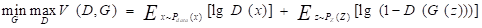(1)

As shown in Eq. 1, x is the real sample set, Pdata (x) is the distribution of the real sample set, z is the noise input into the generator G, and P(Z) is the probability distribution of noise z. Function consists of two parts, the first part represents the input of real data into discriminator D, and maximizes the output entropy to be one. In the second part, the noise data outputs false images through generator G. In other words, the discriminator D tries to maximize V(D, G), while the generator G tries to minimize it.

The false image is imported into discriminator D, which maximizes it to 0, while the generator tries to reduce the difference between the false image and the real image. In other words, the discriminator distinguishes the picture of formula from the false picture generated by generator G(z), and generator G(z) generates the false picture to cheat the discriminator D(x) until real pictures are obtained (Harer et al. 2018). However, the traditional image generated by GAN cannot achieve high resolution, and the obtained dataset is rather fuzzy.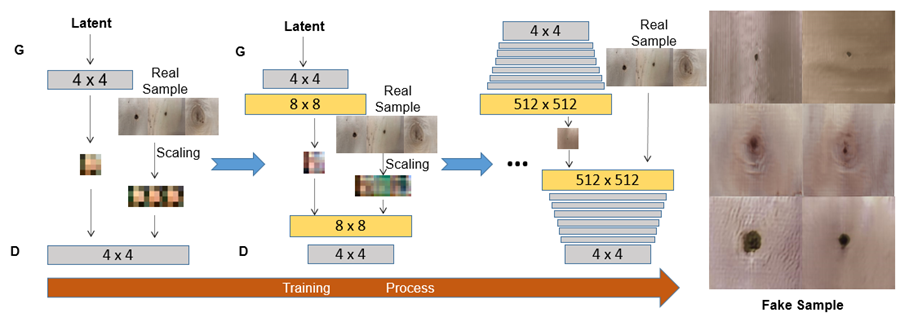Fig. 1. The details of the progressive growing generative adversarial network; the images on the far right are the generated sample images (fake sample)

The image required by the dataset in this paper was a 512 × 512 high-resolution image. Systems, such as DCGAN, WGAN, and other generation adversarial networks, have been unable to meet the requirements. To solve this problem, this paper adopted the PGGAN for image synthesis. The core idea of the algorithm is still to generate images for the confrontation between generator G and discriminator D. In addition, the idea of gradual training from low resolution to high resolution is introduced. In this paper, the training process started from low-resolution (4 × 4) images. Next, layers were gradually added to the network to increase the resolution, until the resolution was increased to 512 × 512 to obtain the training results, and then the training process exited the whole program. The training structure of the neural network (Fig. 1) was drawn.

As the number of layers increases, the system learns the texture details of real samples when training for high resolution. In the process of resolution conversion, the transition is completed by adding smooth layers to reduce the impact of sudden resolution conversion (Togo et al. 2019).

Selection of 350 live knot images was carried out randomly, with 350 dead knot images and 350 insect hole images from the original data. Training data were thereby constructed for image generation. In other words, 1050 defect images were allocated as training data. Then the training step was set to 5000. After the PGGAN expanded the samples, 100 live knot samples, 100 dead knot samples, and 100 insect hole samples were obtained, and the preparation example of wood defect samples are shown in Fig. 2.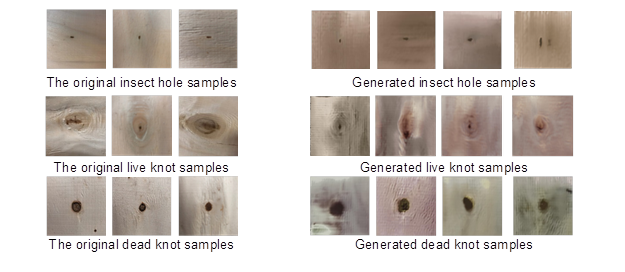Fig. 2. Original samples and generated samples

Each defect shows seven images, of which the three on the left are examples of images captured by the camera, and the four on the right are examples of defects generated by PGGAN. It can be seen that each image generated by PGGAN completely inherits the features of the real image, which can be used as a dataset.

Methods

To reduce the steps of making dataset labels and improve the accuracy of image recognition and classification, this paper adopted the MASK R-CNN algorithm based on transfer learning to identify and classify veneer defects (Yang et al. 2019). MASK R-CNN is an object detection algorithm developed from Faster R-CNN. The purpose of object detection and segmentation is to distinguish different objects in the images and draw a boundary box on the specific object. MASK R-CNN not only can draw a bounding box for the target object, but it can also further mark and classify whether the pixels in the bounding box belong to the object, which can be used to identify the object, mark the boundary of the object, and detect the key points (Nguyen et al. 2018). MASK R-CNN was based on Faster R-CNN, and its application was extended to the field of image segmentation. The process of MASK R-CNN is similar to Faster R-CNN, which uses a Region Proposal Network (RPN) to extract features and classify and tighten boundary boxes (Li et al. 2017). Fast R-CNN adopts RoIPool as the feature extraction method, quantifies each RoI region, and solves the size problem of RoI features of different scales by means of maximum convergence (Behr et al. 2019). However, the process leads to the loss of spatial information, which makes the RoI and extraction features of the original image misplaced (He et al. 2019). MASK R-CNN replaces the RoIPool of Faster R-CNN with RoI alignment (RoIAlign) and continuously uses the RoIAlign of the result object area marked by Mask branch (Qin et al. 2017).

Because there were not many wood defect images, 80% of wood defect images could be taken as the training set and 20% as the validation set. Then, the loss function is: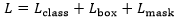. In MASK R-CNN, the most appropriate model was obtained by minimizing the value of the loss function. The trained model was applied for predictive analysis using new data. The loss function of MASK R-CNN was defined as follows: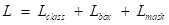(2)

The definition of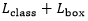is the same as Faster R-CNN,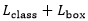is defined as: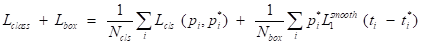(3)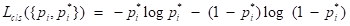(4)

The is the average binary cross-entropy loss: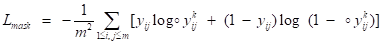(5)

When Mask R-CNN completed defect identification and classification, a large amount of picture data was needed for feature learning. However, collecting wood pictures in the wood industry and manually labeling them cost a lot of manpower and material resources. Therefore, an effective method to adopt Mask R-CNN in the current task was to adopt the strategy of transfer learning.

Considering that modern image classification models have millions of parameters, zero-based training requires a lot of parametric adjustment, as well as a large amount of marker training data and high computational bandwidth. Transfer learning mitigates these requirements by adopting a model that has already been trained on a related task by reusing the learned network. In this paper, the ResNet50 architecture over AlexNet and VGG architectures was chosen. This was because the ResNet50 architecture was more compact than AlexNet and reduces the possibility of overfitting while requiring less computer processing power compared to VGG (Krizhevsky et al. 2017).

The model of MASK R-CNN based on the ResNet50 network structure was established. Through experiments, it was found that the algorithm model of pre-training in the common objects in context (COCO) datasets was migrated to the wood defect dataset for further training, which could achieve accuracy. Only the final full connection layer of the model needs to be modified so that the classifier outputs three values, namely live knot, dead knot, and insect hole. A total of 1600 defect images were collected and generated by PGGAN. Among them, 1280 images were used as a training set and 320 images were used as a validation set. Classification accuracy and confusion matrix were set as the output; the experimental results of MASK R-CNN detection classification were analyzed. In this paper, PyCharm (JetBrains, version 2017.1 Community Edition, Prague, Czech Republic) was used to compile, train, and test on a computer (Lenovo, Beijing, China) with 16 GB of memory, and an i7Core processor with a Titan XP graphics card.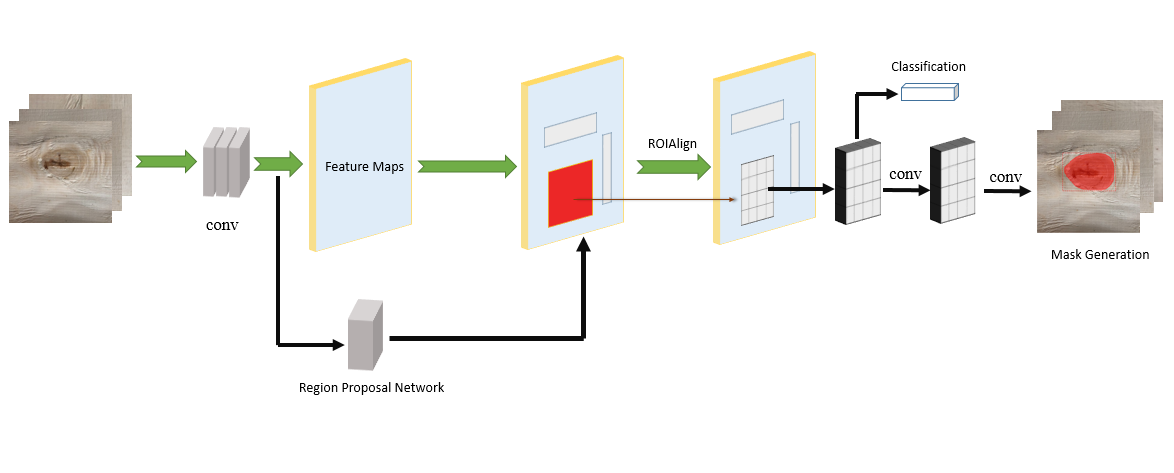Fig. 3. Workflow for identifying wood defects

RESULTS AND DISCUSSION

To test the wood defect detection algorithm based on PGGAN and MASK R-CNN, many experiments were carried out in this work. Wood defect images were 512 × 512 pixels. PGGAN was used to expand the wood defect sample library. The expanded images were used as the training set and transferred the parameters of MASK R-CNN, which had been trained on the COCO dataset into the model to continue the defect dataset for training. Parameter settings of model trainings were set (Table 1).

Table 1. Setting of Model Parameters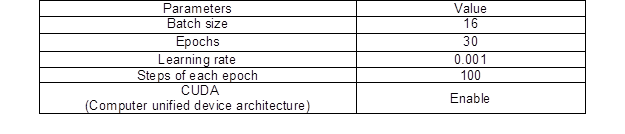To prevent memory explosion, batch training was adopted. The batch size was 16, that is, 16 pictures were extracted from the training set each time for training. The learning rate was set to 0.001, a total of 30 epochs were trained, and each epoch needed to train 100 steps. In other words, the entire model trained 3,000 steps.

Accuracy analysis

At the end of the training, a mathematical model was obtained to detect wood defects. Then, 320 validation set pictures by this model were tested and verified. The performance of the model was evaluated by means of mAP (mean average precision) (Silven et al. 2003). The mAP of a datatset is the average value of each type of AP, and the AP of each type is calculated by the area under the accuracy/recall curve. The specific calculation formula is as follows: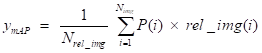(6)

It can be seen from the formula that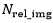is the number of training images with defects detected,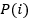is the detection accuracy of the image i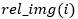determines whether image i is classified correctly. The authors adopted the traditional constant matrix feature and geometry feature as the BP neural network and the characteristics of SOM neural network input by a contrast experiment. The CNN was also used to train and identify the dataset. Different mathematical models were trained and tested in the unexpanded dataset and the dataset expanded by PGGAN. Ten experiments were performed and the average accuracy was recorded. The results of the experiments are shown in Table 2.

Table 2. Comparison of the Accuracy of Different Methods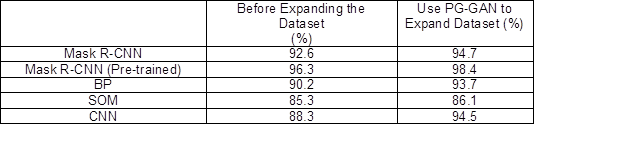Through training 80% of the dataset and testing the remaining 20%, the accuracy of the model trained from scratch on the unexpanded dataset was only able to reach 92.6%, while the accuracy of the model trained on the expanded dataset by PGGAN reached 94.7%.

The application of transfer learning can make the model accuracy of the unexpanded dataset and the expanded dataset reach 96.3% and 98.4%, respectively. The use of PGGAN for detailed dataset expansion and transfer learning can increase the accuracy of the model prediction. However, the experimental results of traditional methods were not satisfactory. The model accuracy of the BP neural network on the unexpanded dataset and the expanded dataset were 90.2% and 93.7%, respectively. The model accuracy of the SOM network in the unexpanded dataset and expanded dataset was 85.3% and 86.1%, respectively. The convolutional neural network also adopted the ResNet50 architecture, and the accuracy on the unexpanded dataset and the expanded dataset was 88.3% and 94.5%, respectively.

Confusion matrix and train loss analysis

Meanwhile, the confusion matrix was used to analyze the experimental results. The abscissa of the confusion matrix is the predicted value of the model for defects, and the ordinate is the real situation of defects (Rojas-Espinoza and Ortiz-Iribarren 2010). Moreover, the accuracy of each kind of prediction can be analyzed according to the confusion matrix, which shows the imbalance of samples. The loss function of MASK R-CNN is composed of three parts. The change of the loss function and its composition in the training process of the model are shown (Fig. 4).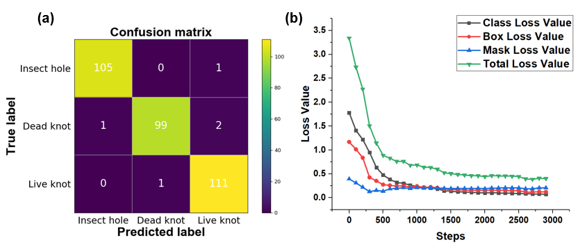Fig. 4. (a) Confusion matrix of wood defect classification and (b) loss plot during training

It can be seen from the confusion matrix that the validation set contained 106 insect holes, 102 dead knots, and 112 live knots. According to the prediction results of the model, the prediction accuracy of insect hole, dead knot, and live knot was 99.05%, 97.05%, and 99.10%, respectively. In other words, the mAP was 98.4%. It can be seen that the expanded dataset with PGGAN had a great improvement in defect identification based on MASK R-CNN under the strategy of transfer learning compared with the traditional classification method.

As shown in Fig. 4b, after adopting the strategy of transfer learning, the change of the total loss value was divided into three stages: (1) the loss value of the first 500 steps declined rapidly; (2) the loss value of steps from 500 to 1500 declined slowly; (3) step tended to be stable from 1500 to 3000 times. The total loss value of the model was stable at 0.4003.